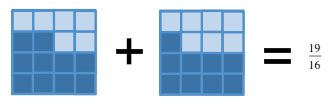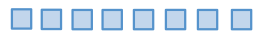### Home > CC2 > Chapter Ch2 > Lesson 2.3.1 > Problem2-117

2-117.

Copy each expression and then simplify it without using a calculator. Be sure to show all steps.1. $7\frac{5}{8}+2\frac{9}{16}$

This sum can be represented with a diagram: Diagram expression with 2 equal sized rectangles: Left Rectangle + Right Rectangle. Left rectangle, 4 rows of 2 columns, with 5 shaded. Right Rectangle, 4 rows of 4 columns, with 9 shaded.

Find a common denominator to add the two fractions. Changes: additional columns added to left rectangle, to make it 4 rows of 4 columns, with 10 shaded.

Changes: After right rectangle, an equal sign and the fraction 19 sixteenths.2. $2\frac{7}{8}+6\frac{1}{6}$

See part (a) for strategy.

3. $8-6\frac{2}{5}$

Draw $8$ squares on your paper like this: 8 square tiles.Cross off $6\frac{2}{5}$. What is left?

$1\frac{3}{5}$